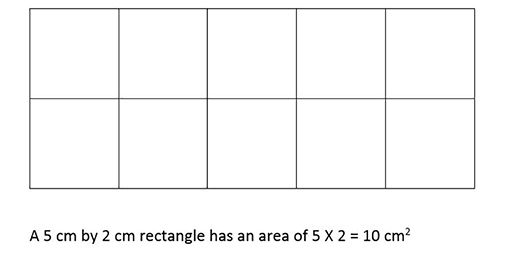Teaching mathematics

Start this free course now. Just create an account and sign in. Enrol and complete the course for a free statement of participation or digital badge if available.

Free course

# 4.1 Area

It is best to introduce the topic of area by considering the inside of shapes as being divided into unit squares with area being recorded as the number of squares and the units of area (e.g. 10 cm2). In contrast, the perimeter of a shape is the total length of its boundary but this is often confused with area by learners.

The next stage in learning about area is to find the area of rectangles which have sides measured as whole numbers. It is straightforward for learners to note that the rectangles contain an array of unit squares (Figure 12). This leads to the general formula for the area of a rectangle = length × width.Figure 12 A 5 cm by 2 cm rectangle divided into square units
TM_1